# AP Board 3rd Class Maths Solutions 6th Lesson Let’s Share

Andhra Pradesh AP Board 3rd Class Maths Solutions 6th Lesson Let’s Share Textbook Exercise Questions and Answers.

## AP State Syllabus 3rd Class Maths Solutions Chapter 6 Let’s Share

Textbook Page No. 75

I. Colourful bouquets:
“Malli” lives in a village. Her father Yadayya has a nursery. He gave her 18 roses and told to make bouquets with 6 roses in each one. Malli started preparing bouquets. How many bouquets can Malli make?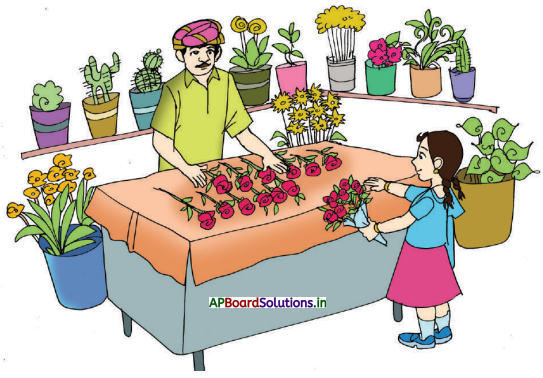Question 1.
How many roses are given to Malli to make bouquets ?
18 roses

Question 2.
How many roses should be used to make one bouquet ?
6 rosesQuestion 3.
How many number of bouquets can she make ?
18 ÷ 6 = 3 bouquets

Textbook Page No. 90

Do these

Do the following divisions and find out the dividend, divisor, quotient and remainder.

1. 30 ÷ 6,
Dividend = ____,
Divisor = ____
Quotient = ____,
Remainder = _____2. 30 ÷ 5
Dividend = _____,
Divisor = ____,
Quotient = ___,
Remainder = ____

Textbook Page No. 78

Do these

Question 1.
Write the division facts for the following multiplication facts.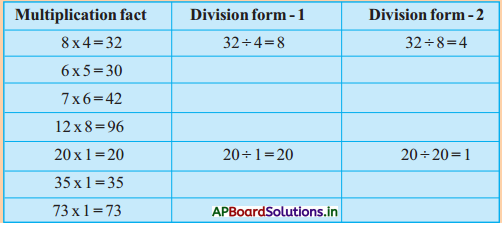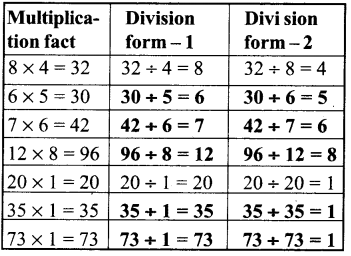Textbook Page No. 79

II. Making word problems:

Example: 30 ÷ 6 = 5
30 ÷ 5 = ?
If 30 laddoos are distributed to 5 persons equally, how many laddoos will each person get?Observe the pictures and fill the blank.

1) 24 ÷ 4 = ?
If 24 _____ are distributed among ____ persons equally.
how many ___ will each person get ?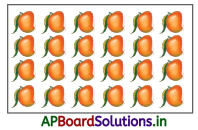If 24 Mangoes are distributed among 4 persons equally.
how many Mangoes will each person get ?

2) 12 ÷ 3 = ?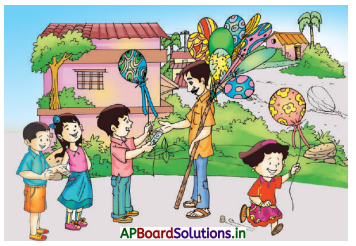12 _____ are distributed among ____ children equally.
How many _____ will each child get?
12 balloons are distributed among 4 children equally.
How many balloons  will each child get?3) 20 ÷ 4 = ?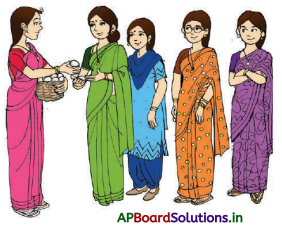If an anganwadi teacher is distributing 20 ____ to ____   mothers, how many _____ will each get ?
If an anganwadi teacher is distributing 20 eggs to 4 mothers, how many eggs will each get ?

Textbook Page No. 80

Question 4.
Write your own problem for division fact 12 ÷ 4 = 3
12 chocolates are distributed among 4 children equally. How many chocolates will each child get?

Textbook Page No. 82

Do these:

Question 1.
55 ÷ 5 = ____
A. Dividend = _____
Divisor = _____
Quotient = ____
Remainder = ____

Question 2.
84 tyres were put on cars. If each car needs 4 tyres, how many cars would be fitted with tyres ?
Number of tyres availabe =
Number of tyres needed for 1 car =
Number of cars fitted with tyres = ____ ÷ ____
Number of tyres availabe = 84
Number of tyres needed for 1 car = 4
Number of cars fitted with tyres = 84 ÷ 4
= 21 cars

Question 3.
If 92 are distributed among 4 children, how much money does each child get ?
23Question 4.
64 ÷ 8 = _____
Dividend = ____,
Divisor = ____,
Quotient = ____
Remainder = ____

Question 5.
63 children were standing in 9 rows equally How many children were in each row ?
Number of children = ____
Number of rows = ____
Children standing in each row = 63 ÷ 9
= 7

Textbook Page No. 84

Do these:

Question 1.
Harish has 98 plants with him. He wants to distribute them equally among 6 schools, how many plants does each school get ? How many are left over ?
Number of plants with Harish = 98
Number of schools = 6
Plants each school gets = 98 ÷ 6
Plants leftover = 2

Question 2.
Ramana distributed Rs. 70 among 4 children. How much money does each child get? How many rupees are leftover ?
Amount to be distributed (in rupees) = 70
Number of children = 4
Each child gets = 70 ÷ 4
Number of rupees left over = 2

Question 3.
There are 65 students in a school. The teacher wants to make them stand in 8 rows. He did like that. What is your observation ?
Total number of students = 65
Number of rows to be formed = 8
Number of rows formed = 65 ÷ 8 = 8
Number of students remained = 1

4. Fill the following blanks.

1.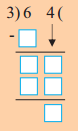Dividend = ___
Divisor = ____
Quotient = ____
Remainder = ____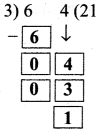Dividend = 64
Divisor = 3
Quotient = 21
Remainder = 1

2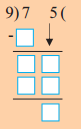Dividend = ___
Divisor = ____
Quotient = ____
Remainder = ____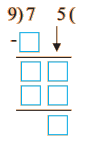Dividend = 64
Divisor = 3
Quotient = 21
Remainder = 1

3.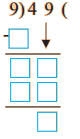Dividend = ___
Divisor = ____
Quotient = ____
Remainder = ____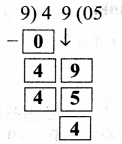Dividend = 49
Divisor = 9
Quotient = 5
Remainder = 4

Textbook Page No. 86

Do these:

1. 380 ÷ 3 = _____
126

2. 306 ÷ 6 = ____
51

Question 3.
The strength of a school is 695. If 5 students can sit on one bench, how many benches are required?
Strength of the school =
Number of students sitting on one bench =
Number of benches required =
Strength of the school = 695
Number of students sitting on one bench = 5
Number of benches required = 695 ÷ 5 = 139Question 4.
9 oranges can be packed in a box. How many boxes will be needed to pack 738 oranges?
Number of oranges in a box = 9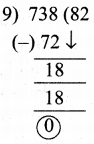Number of oranges = 738 18
Number of required boxes 18
= 738 ÷ 9
= 82 boxes

Question 5.
700 students are divided into 6 groups. How many students are there in each group? How many are left over?
Number of students = 700
Number of groups = 6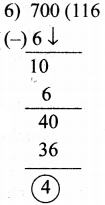Number of students in each group = 700 ÷ 6
= 116 groups
Left members 4 members

I. Even numbers and Odd numbers:

I. Observe the following division: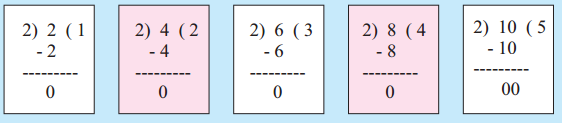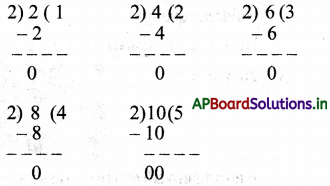* What is the remainder in all the above divisions? _____
zero

* It means that 2,4,6,8 and 10 are exactly divisible by _____
2

* Such numbers are called EVEN numbers.
Any number that can be divided exactly by 2 is called an even number.

II.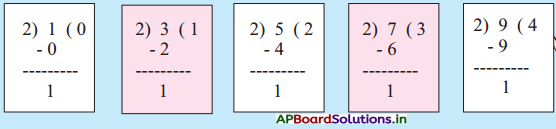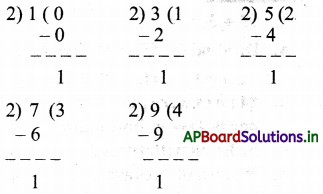* What is the remainder in all the above divisions? _____
One

* It means that 1,3,5,7,9 are not exactly divisible by 2

* Such numbers are called ODD numbers.
Any number that can not be divided exactly by 2 is called an odd number.

Textbook Page No. 87

Activity :

1 to 30 numbers are given below. Circle the even numbers.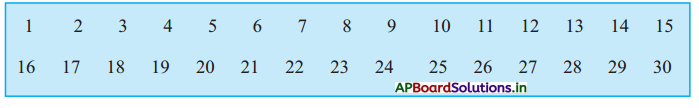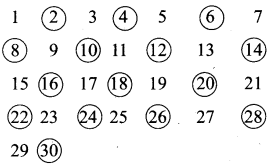* Write circled numbers
even numbers

* Write un circled numbers
odd numbers

* Write digits in ones place of even numbers (circled)
2, 4, 6, 8, 10, 12, 14, 16, 18, 20, 22, 24, 26, 28, 30

* Write digits in ones place of odd numbers (uncircled)
1, 3, 5, 7, 9, 11, 13, 15, 17, 19, 21, 23, 25, 27, 29

* What do you observe ?
* Odd numbers have 1, 3, 5, 7 or 9 in their ones place

* Even numbers have 0, 2, 4, 6, or 8 in their ones place

Try these:

a) Write the next even number.
1) 38, ____
40

2) 46, ____
483) 84, ____
86

b) Write the next odd number

1) 135, ___
137

2) 847, ___
849

3) 965, ____
967

c) Which of the following are even numbers ? Why ?
1) 784
2) 835
3) 963
784

d) Which of the following are odd numbers ? Why ?
1) 645
2) 237
3) 840
237

Exercise

1. Fill in the blanks.

a) 55 ÷ 55 = ____

b) 175 ÷ 5 = ____

c) 12 × 13 = 156 so ____ ÷ ____ = _____ and _____ ÷ _____ = _____
so 156 ÷ 12 = 13 and 156 ÷ 13 = 12

d) 25 × 20 = 500 so ____ ÷ ____ = _____ and _____ ÷ _____ = _____
so 500 ÷ 25 = 20 and 20 and 500 ÷ 20 = 25

Question 2.
Divide and write dividend, divisor, quotient and remainder
a) 60 ÷ 5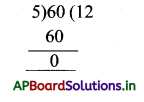Dividend = 60
Divisor = 5
Quotient = 12
Remainder = 0b) 79 ÷ 8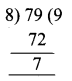Dividend = 79
Divisor = 8
Quotient = 9
Remainder = 7

c) 150 ÷ 6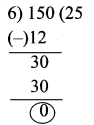Dividend = 150
Divisor = 6
Quotient = 25
Remainder = 0

d) 220 ÷ 4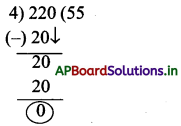Dividend = 220
Divisor = 4
Quotient = 55
Remainder = 0

e) 496 ÷ 7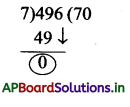Dividend = 496
Divisor = 7
Quotient = 70
Remainder = 6

f) 589 ÷ 9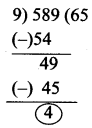Dividend = 589
Divisor = 9
Quotient = 65
Remainder = 4

g) 380 ÷ 3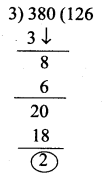Dividend = 380
Divisor = 3
Quotient = 126
Remainder = 2

h) 940 ÷ 2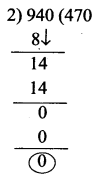Dividend = 940
Divisor = 2
Quotient = 470
Remainder = 0Question 3.
One jug of water can fill 7 tumblers. How many jugs of water are needed to fill 84 tumblers?
One jug = 7 tumblers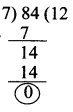Number of tumblers = 84
Number of jugs = 84 ÷ 7
= 12 jugs

Question 4.
There are 7 days in a week and 365 days in a year. How many weeks are there in a year? How many extra days are there ?
Number of days in a week = 7
Number of days in a year = 365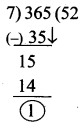Number of weeks in a year = 365 ÷ 7
Number of weeks in a year = 52
Number of extra days = 1

Question 5.
Write all the even numbers between 760 to 800
All even numbers between 760 to 800 = 762, 764, 766, 768, 770, 772, 774, 776, 778, 780, 782, 784, 786, 788, 790, 792, 794, 796, 798

Question 6.
Write all the odd numbers between 860 to 900.
All odd numbers between 860 to 900 = 861, 863, 865, 867, 869, 871, 873, 875, 877, 879, 881, 883, 885, 887, 889, 891, 893, 895, 897, 899

Question 7.
Classify the following as even and odd numbers.
a) 396
b) 495
c) 893
d) 747
e) 898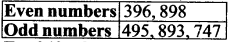Question 8.
For 240 ÷ 8, create a word problem. In a cinema theatre, 8 people can sit in a row in how many rows can 240 people sit ?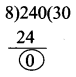240 ÷ 8 = 30 rows.

Question 9.
There are 54 gulab jamuns in a vessel. These are to be distributed equally among 9 girls, how many gulab jamuns should each girl get?
Number of gulabjamuns in a vessel = 54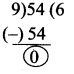Number of girls = 9 members
Number of gulabjamuns each girl get = 54 ÷ 9 = 6jamunsQuestion 10.
The cost of 9 mangoes is Rs. 45. Find the cost of one mango?
Number of mangoes = 9
Cost of 9 mangoes = ₹ 45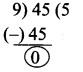Costofeachmango = 45 ÷ 9 = ₹ 5

Question 11.
4 students can sit on one bench. On how many benches can 36 boys sit?
Number of students can sit in one bench = 4
Number of students totally = 36
Required number of benches = 36 ÷ 4 = 9 benches

Question 12.
If we cut 40 metre ribbon into 9 pieces, what is the length of each piece ?
Length of the ribbon = 40 m
Number of pieces = 9
Length of each piece = 40 ÷ 9 = 4 m

Question 13.
How many tri cycles can be made using 72 wheels ?
Total number of wheels = 72
Number of wheels to each tri cycle = 3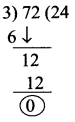Number of tri cycles = 72 ÷ 3
= 24 cycles

Question 14.
The product of two numbers is 168. If one of them is 4, And the other number ?
Let the number be = x
One number be = 4
Product of two numbers = 168
4x = 168
x = 168 ÷ 4
Other number = x = 42

Question 15.
225 school children are to be divided into 5 groups. How many children are there in each group?
Number of school children = 225
Number of groups = 5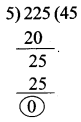Number of children in each group = 225 ÷ 5
No.of students in each group = 45 students

Question 16.
640 Kg of rice is shared equally among 6 persons. Find how many Kgs of rice will each one get and how many Kgs of rice will be left?
Totalquantityofnce = 640 Kg
Number of persons = 6
Number of Kgs, each person will get
= 640 ÷ 6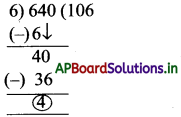Each one will get = 106 Kgs ;
Left rice quantity = 4 Kgs

Multiple Choice Questions

Question 1.
Choose the symbol which represents division ( )
A) +
B) –
C) ×
D) ÷
D) ÷Question 2.
When a number is divided by 1, we get the ….. number. ( )
A) Opposite
B) Same
C) Negative
D) Positive
B) Same

Question 3.
The number which is to be divided by another number is called ( )
A) Dividend
B) Divisor
C) Quotient
D) Remainder
A) Dividend

Question 4.
The number which divides another number is called ( )
A) Dividend
B) Divisor
C) Quotient
D) Remainder
B) Divisor

Question 5.
The result we get after the division ()
A) Dividend
B) Divisor
C) Quotient
D) Remainder
C) Quotient

Question 6.
The number which remains after division ( )
A) Dividend
B) Divisor
C) Quotient
D) Remainder
D) Remainder

Question 7.
Any number that can be divided exactly by ‘2’ is called …… number. ( )
A) even
B) odd
C) both A and B
D) None